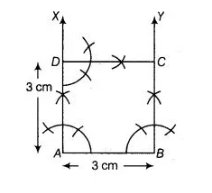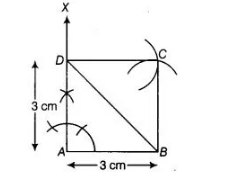# Construct a square of side 3 cm.`
Question:

Construct a square of side 3 cm.

Thinking Process

Firstly, draw a line segment of given length. In both ends of segment draw an angle of 90° from these line segment draw a line upto the given length.

Further draw an angle of 90° from these line and join the other line, to get the required construction.

Solution:

We know that, each angle of a square is right angle (i.e., 90°).
To construct a square of side 3 cm, use the following steps.

1. Draw a line segment AS of length 3 cm.
2. Now, generate an angle of 90° at points A and B of the line segment and plot the parallel lines AX and BY at these points.
3. Cut AD and SC of length 3 cm from AX and BY, respectively.
4. Draw an angle of 90° at any one of the point C or D and join both points by a line segment CD of length 3 cm.
Thus, ABCD is the required square of side, 3 cm.Alternate Method

To construct a square $A B C D$ of side $3 \mathrm{~cm}$, use the following steps

1. Draw a line segment $A B$ of length $3 \mathrm{~cm}$.

2. Now, draw an angle $X A B=90^{\circ}$ at point $A$ of line segment $A B$.

3. Cut a line segment $A D=3 \mathrm{~cm}$ from the ray $A X$ and join $B D$.

4. Now, from $D$ point $C$ is at a distance of $3 \mathrm{~cm}$. So, having $D$ as centre, draw an arc of radius $3 \mathrm{~cm}$.5. From B, point $C$ is at a distance of $3 \mathrm{~cm}$. So, having 8 as centre, draw an arc of radius $3 \mathrm{~cm}$ which intersect previous arc (obtained in step iv) at $\mathrm{C}$.

6. Join $D C$ and $B C$. Thus, $A B C D$ is the required square of side $3 \mathrm{~cm}$.